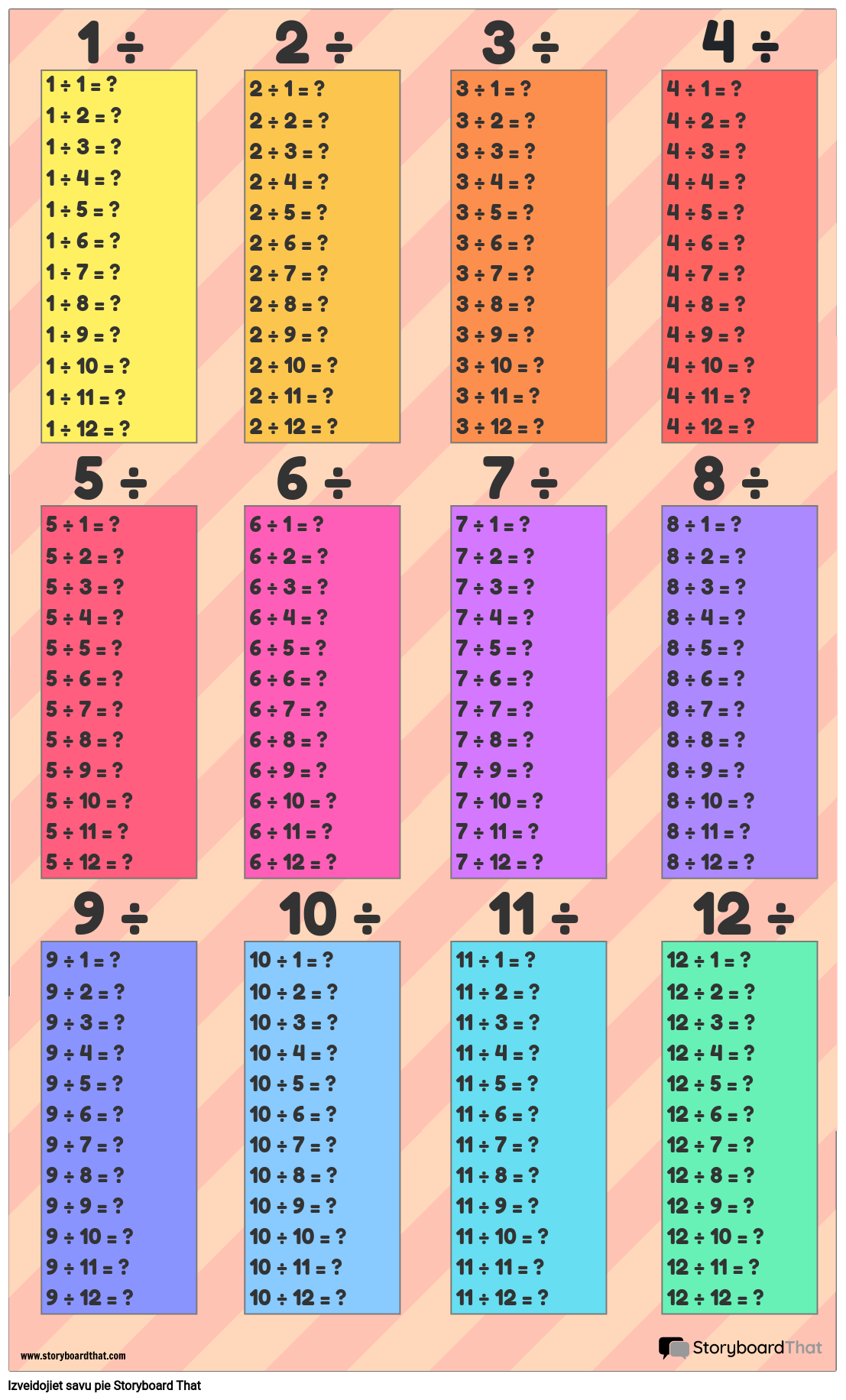### Izveidojiet savu Storyboard

Izmēģiniet to bez maksas!### Izveidojiet savu Storyboard

Izmēģiniet to bez maksas!

#### Montāžas Apraksts

Izveidojiet dalīšanas galda plakātus savai klasei!

#### Montāžas Teksta

• ﻿﻿﻿﻿﻿﻿﻿﻿﻿﻿1 ÷ 1 = ﻿﻿﻿?1 ÷ 2 = ?1 ÷ 3 =﻿﻿﻿ ?1 ÷ 4 = ? 1 ÷ 5 =﻿﻿﻿ ? 1 ÷ 6 = ? 1 ÷ 7 =﻿﻿ ? 1 ÷ 8 = ? 1 ÷ 9 = ? 1 ÷ 10 = ? 1 ÷ 11 = ? 1 ÷ 12 = ?
• ﻿﻿﻿﻿﻿﻿﻿﻿﻿﻿﻿5 ÷ 1 =﻿﻿﻿ ? 5 ÷ 2 = ? 5 ÷ 3 =﻿﻿﻿ ? 5 ÷ 4 = ? 5 ÷ 5 =﻿﻿﻿ ? 5 ÷ 6 = ? 5 ÷ 7 =﻿﻿﻿ ? 5 ÷ 8 = ? 5 ÷ 9 = ? 5 ÷ 10 = ? 5 ÷ 11 = ? 5 ÷ 12 = ?
• ﻿﻿﻿﻿﻿﻿﻿1 ÷
• ﻿﻿﻿﻿﻿﻿﻿﻿﻿﻿5 ÷
• ﻿﻿﻿﻿﻿﻿﻿﻿﻿﻿﻿﻿﻿2 ÷ 1 =﻿﻿﻿ ? 2 ÷ 2 = ? 2 ÷ 3 =﻿﻿﻿ ? 2 ÷ 4 = ? 2 ÷ 5 =﻿﻿﻿ ? 2 ÷ 6 = ? 2 ÷ 7 =﻿﻿﻿ ? 2 ÷ 8 = ? 2 ÷ 9 = ? 2 ÷ 10 = ? 2 ÷ 11 = ? 2 ÷ 12 = ?
• ﻿﻿﻿﻿﻿﻿﻿﻿﻿﻿﻿﻿﻿﻿6 ÷ 1 =﻿﻿﻿ ? 6 ÷ 2 = ? 6 ÷ 3 =﻿﻿﻿ ? 6 ÷ 4 = ? 6 ÷ 5 =﻿﻿﻿ ? 6 ÷ 6 = ? 6 ÷ 7 =﻿﻿﻿ ? 6 ÷ 8 = ? 6 ÷ 9 = ? 6 ÷ 10 = ? 6 ÷ 11 = ? 6 ÷ 12 = ?
• ﻿﻿﻿﻿﻿﻿﻿﻿﻿﻿2 ÷
• ﻿﻿﻿﻿﻿﻿﻿﻿﻿﻿﻿﻿6 ÷
• ﻿﻿﻿﻿﻿﻿﻿﻿﻿﻿﻿﻿﻿﻿3 ÷ 1 =﻿﻿﻿ ? 3 ÷ 2 = ? 3 ÷ 3 =﻿﻿﻿ ? 3 ÷ 4 = ? 3 ÷ 5 =﻿﻿﻿ ? 3 ÷ 6 = ? 3 ÷ 7 =﻿﻿﻿ ? 3 ÷ 8 = ? 3 ÷ 9 = ? 3 ÷ 10 = ? 3 ÷ 11 = ? 3 ÷ 12 = ?
• ﻿﻿﻿﻿﻿﻿﻿﻿﻿﻿﻿﻿﻿﻿﻿﻿﻿﻿7 ÷ 1 =﻿﻿﻿ ? 7 ÷ 2 = ? 7 ÷ 3 =﻿﻿﻿ ? 7 ÷ 4 = ? 7 ÷ 5 =﻿﻿﻿ ? 7 ÷ 6 = ? 7 ÷ 7 =﻿﻿﻿ ? 7 ÷ 8 = ? 7 ÷ 9 = ? 7 ÷ 10 = ? 7 ÷ 11 = ? 7 ÷ 12 = ?
• ﻿﻿﻿﻿﻿﻿﻿﻿﻿﻿﻿﻿3 ÷
• ﻿﻿﻿﻿﻿﻿﻿﻿﻿﻿﻿﻿﻿7 ÷
• ﻿﻿﻿﻿﻿﻿﻿﻿﻿﻿﻿﻿﻿﻿﻿﻿﻿﻿﻿﻿4 ÷ 1 =﻿﻿﻿ ? 4 ÷ 2 = ? 4 ÷ 3 =﻿﻿﻿ ? 4 ÷ 4 = ? 4 ÷ 5 =﻿﻿﻿ ? 4 ÷ 6 = ? 4 ÷ 7 =﻿﻿﻿ ? 4 ÷ 8 = ? 4 ÷ 9 = ? 4 ÷ 10 = ? 4 ÷ 11 = ? 4 ÷ 12 = ?
• ﻿﻿﻿﻿﻿﻿﻿﻿﻿﻿﻿﻿﻿﻿﻿﻿﻿﻿8 ÷ 1 =﻿﻿﻿ ? 8 ÷ 2 = ? 8 ÷ 3 =﻿﻿﻿ ? 8 ÷ 4 = ? 8 ÷ 5 =﻿﻿﻿ ? 8 ÷ 6 = ? 8 ÷ 7 =﻿﻿﻿ ? 8 ÷ 8 = ? 8 ÷ 9 = ? 8 ÷ 10 = ? 8 ÷ 11 = ? 8 ÷ 12 = ?
• ﻿﻿﻿﻿﻿﻿﻿﻿﻿﻿﻿﻿﻿4 ÷
• ﻿﻿﻿﻿﻿﻿﻿﻿﻿﻿﻿﻿﻿4 xClick To Edit﻿﻿﻿﻿﻿﻿﻿﻿﻿﻿﻿﻿﻿﻿4 ÷HelpEscapeInsert ParagraphUndo the last commandRedo the last commandTabUntabSet a bold styleSet a italic styleSet a underline styleSet a strikethrough styleClean a styleSet left alignSet center alignSet right alignSet full alignToggle unordered listToggle ordered listOutdent on current paragraphIndent on current paragraphChange current block's format as a paragraph(P tag)Change current block's format as H1Change current block's format as H2Change current block's format as H3Change current block's format as H4Change current block's format as H5Change current block's format as H6Insert horizontal ruleShow Link DialogSummernote 0.8.18 · Project · Issues
• ﻿﻿﻿﻿﻿﻿﻿﻿﻿﻿﻿﻿﻿﻿﻿8 ÷
• ﻿﻿﻿﻿﻿﻿﻿﻿﻿﻿﻿﻿9 ÷ 1 =﻿﻿﻿ ? 9 ÷ 2 = ? 9 ÷ 3 =﻿﻿﻿ ? 9 ÷ 4 = ? 9 ÷ 5 =﻿﻿﻿ ? 9 ÷ 6 = ? 9 ÷ 7 =﻿﻿﻿ ? 9 ÷ 8 = ? 9 ÷ 9 = ? 9 ÷ 10 = ? 9 ÷ 11 = ? 9 ÷ 12 = ?
• ﻿﻿﻿﻿﻿﻿﻿﻿﻿﻿9 ÷
• ﻿﻿﻿﻿﻿﻿﻿﻿﻿﻿﻿﻿﻿﻿10 ÷ 1 =﻿﻿﻿ ? 10 ÷ 2 = ? 10 ÷ 3 =﻿﻿﻿ ? 10 ÷ 4 = ? 10 ÷ 5 =﻿﻿﻿ ? 10 ÷ 6 = ? 10 ÷ 7 =﻿﻿﻿ ? 10 ÷ 8 = ? 10 ÷ 9 = ? 10 ÷ 10 = ? 10 ÷ 11 = ? 10 ÷ 12 = ?
• ﻿﻿﻿﻿﻿﻿﻿﻿﻿﻿﻿10 ÷
• ﻿﻿﻿﻿﻿﻿﻿﻿﻿﻿﻿﻿﻿﻿﻿﻿11 ÷ 1 =﻿﻿﻿ ? 11 ÷ 2 = ? 11 ÷ 3 =﻿﻿﻿ ? 11 ÷ 4 = ? 11 ÷ 5 =﻿﻿﻿ ? 11 ÷ 6 = ? 11 ÷ 7 =﻿﻿﻿ ? 11 ÷ 8 = ? 11 ÷ 9 = ? 11 ÷ 10 = ? 11 ÷ 11 = ? 11 ÷ 12 = ?
• ﻿﻿﻿﻿﻿﻿﻿﻿﻿﻿﻿﻿﻿11 ÷
• ﻿﻿﻿﻿﻿﻿﻿﻿﻿﻿﻿﻿﻿﻿﻿﻿﻿﻿12 ÷ 1 =﻿﻿﻿ ? 12 ÷ 2 = ? 12 ÷ 3 =﻿﻿﻿ ? 12 ÷ 4 = ? 12 ÷ 5 =﻿﻿﻿ ? 12 ÷ 6 = ? 12 ÷ 7 =﻿﻿﻿ ? 12 ÷ 8 = ? 12 ÷ 9 = ? 12 ÷ 10 = ? 12 ÷ 11 = ? 12 ÷ 12 = ?
• ﻿﻿﻿﻿﻿﻿﻿﻿﻿﻿﻿﻿﻿﻿12 ÷
Izveidoti vairāk nekā 30 miljoni stāstu shēmu